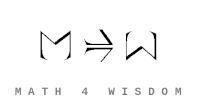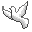Community Discussion list Contact Andrius Kulikauskas m a t h 4 w i s d o m @ g m a i l . c o m +370 607 27 665 Eičiūnų km, Alytaus raj, LithuaniaMy original work is in the Public Domain for you to use in your best judgement. Thank you for your support! Patreon Paypal to ms@ms.ltBookshelfAs an Amazon Associate I earn from qualifying purchases. Thank you! Upload Adjoint strings I am trying to classify adjoint strings and relate them to divisions of everything or their representations. An adjoint string of {$N$} functors {$F_0 \dashv F_1 \dashv \cdots \dashv F_{N-2} \dashv F_{N-1}$} is one for which {$F_0$} has no left adjoint functor and {$F_{N-1}$} has no right adjoint functor. Adjunction is a relationship that distinguishes a left adjoint functor {$F:\mathcal{D}\rightarrow\mathcal{C}$} and a right adjoint functor {$G:\mathcal{C}\rightarrow\mathcal{D}$}. Evidently, it is a profoundly meaningful relationship which manifests itself in a wide variety of examples. In each case, the left adjoint and the right adjoint play different conceptual roles which can't be switched around. Thus adjunction can ground and express the distinction of roles. A functor {$F$} may or may not have a right adjoint. But if it does have a right adjoint, then that right adjoint is unique up to isomorphism. Similarly with left adjoints. We get strings of adjoints of various lengths, but nothing more complicated, that is, no branches. Conceptually, examples of adjoint strings can be grouped into families that illustrate the same theme. Thus I am collecting and grouping examples and trying to understand their conceptual underpinnings. Adjoint strings of length {$N$} may express conceptual relationships between {$N$} perspectives and thus may indeed express divisions of everything. For example, the adjunction between a free construction {$F$} and a forgetful functor {$G$} may model the division of everything into two perspectives, as with free will and fate. I also find it noteworthy that, in the case of abelian categories {$\mathcal{C}$} and {$\mathcal{D}$}, every left adjoint functor {$F$} is right-exact, which means that if {$0\rightarrow D_1\rightarrow D_2\rightarrow D_3\rightarrow 0$} is exact, then {$F(D_3)\rightarrow F(D_2)\rightarrow F(D_1)\rightarrow 0$} is exact. Similarly, every right adjoint functor {$G$} is left-exact, which means that if {$0\rightarrow C_1\rightarrow C_2\rightarrow C_3\rightarrow 0$} is exact, then {$0\rightarrow F(D_3)\rightarrow F(D_2)\rightarrow F(D_1)$} is exact. (See: Exact functor and Exact sequence.) I have previously considered that exact sequences from {$0$} to {$0$} of length {$N$} may express divisions of everything of length {$N-2$}, so I find the connection worth considering. Adjoint strings may perhaps express the relevant concepts more abstractly. One potential problem with adjoint strings is that the functors take us back and forth between {$\mathcal{D}$} and {$\mathcal{C}$}. It's not clear to me what that would mean for divisions of everything. However, it is possible that {$\mathcal{D} = \mathcal{C}$}, so that the functors {$F$} and {$G$} are endofunctors. That would drastically reduce the number of examples but perhaps the conceptual ideas would persist. Another issue is that conceptually there seem to be several different families of adjoint strings having the same length, for example, length {$2$}. Thus adjoint strings may perhaps express not divisions themselves but rather their representations. For example, Grothendieck's six operations comprise an adjoint string of length {$2$} and an adjoint string of length {$4$} which seem to express the six representations. Again, if the free-forgetful adjunction expresses free will vs. fate, then that is but one of four representations of the division of everything into two perspectives. Research pages
Šis puslapis paskutinį kartą keistas January 17, 2021, at 03:34 PM